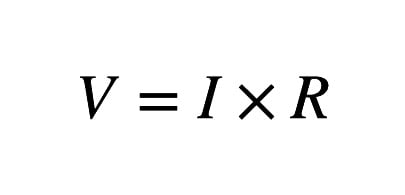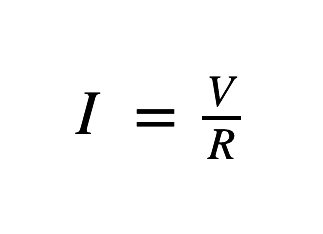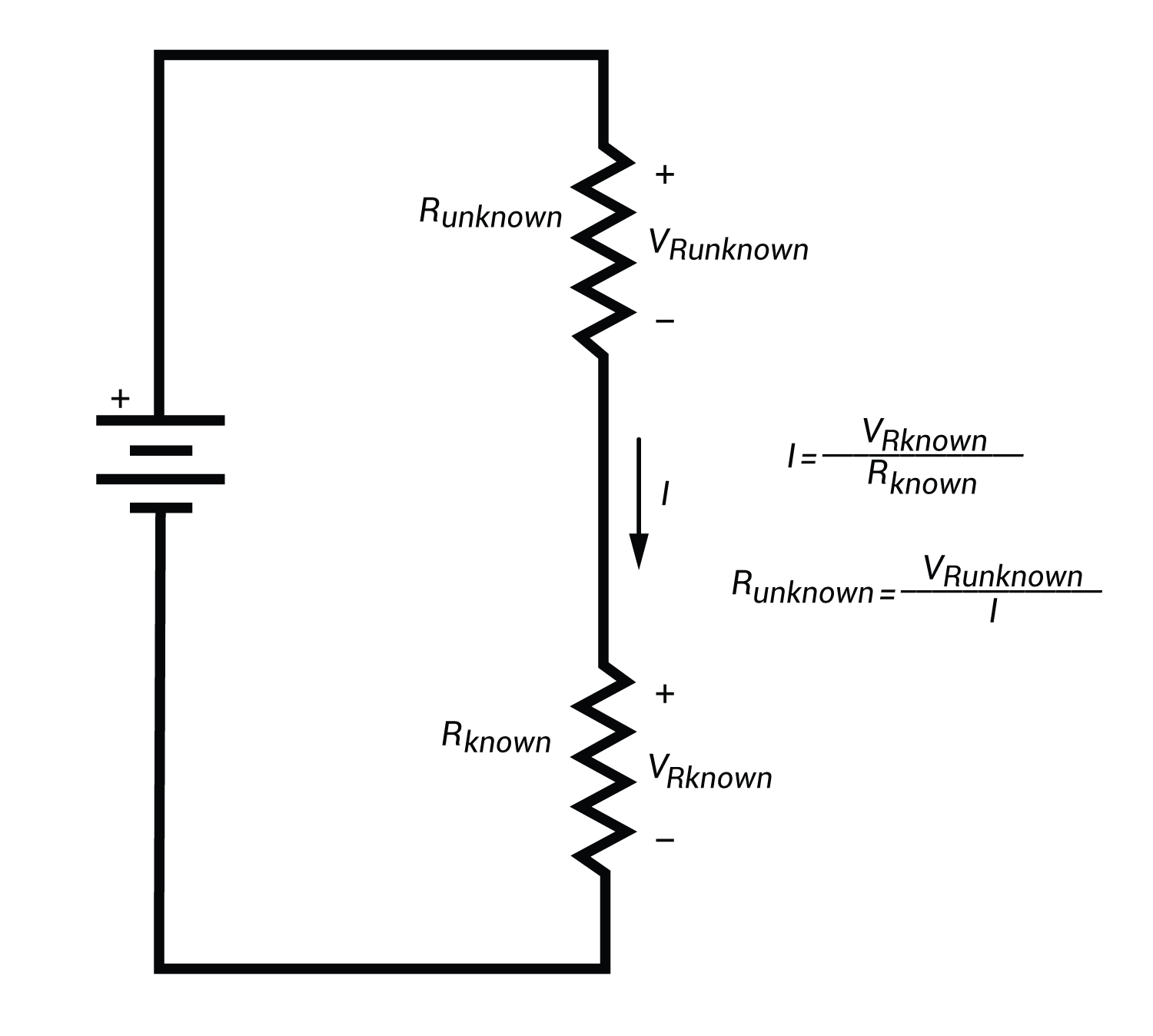Technical Article

# Measuring Resistance, In Circuit and Out

June 21, 2015 by Robert Keim

## This article explains how to measure a resistance value, even if the resistor cannot be removed from its circuit.

The resistor is the fundamental electronic component. By resisting the flow of electrons in a simple and predictable way, a resistor allows the designer to easily manipulate currents and voltages—and currents and voltages are what circuits are all about.

Beginner

#### Before You Measure

The resistance, or simply the “value” of a resistor determines how it will influence the circuit to which it is connected. You need to know the resistance of your resistor—sometimes the approximate value is fine--but sometimes you need precision. The value of a resistor is usually indicated on the component itself, with either old-fashioned colored bands or printed numerals. But these are nominal values, meaning that the actual resistance can be a certain percentage higher or lower than this indicated value. If the tolerance of the resistor is 10%, for example, a “1000 ohm” resistor could actually be anywhere between 900 and 1100 ohms.

#### Why Measure?

So if the resistance value is labeled right there on the resistor, why would you need to measure? There are two reasons: first, you may not be able to confidently determine the resistance from the label—maybe the component is old and the label is faded, or maybe you don’t understand the color code. Second, you may need to know the exact value of a specific resistor, not the nominal value. A high-precision circuit requires high-precision components. If the reference voltage for an analog-to-digital converter is determined by an external resistor, you need to know the exact value of that resistor in order to accurately interpret your digitized measurements.

#### Know the Law

The most basic law describing the flow of electric current is Ohm’s law, which relates voltage (V) and current (I) to resistance (R):In other words, the voltage supplied to a circuit is equal to the current flowing through the circuit multiplied by the total resistance of the circuit. Another way to express this same relationship isThis means that the current flowing through a circuit is equal to the voltage supplied to the circuit divided by the total resistance of the circuit.

Ohm’s law applies not only to entire circuits but also to individual components. With a resistor, energy is dissipated as current flows through the resistive material, and this energy loss is manifested as a voltage drop, which is the difference between the voltages at the two terminals of the resistor. Thus, Ohm’s law provides the essential approach to measuring the value of a resistor: if you know the voltage drop across the resistor and the current flowing through the resistor, you know the resistance.

#### The Easy Way

The most common and simplest way to measure resistance is with a digital multimeter, or DMM. This indispensable device knows all about Ohm’s law and is happy to do the work for you: when you connect the terminals of the resistor to the two probes, it supplies a known current, measures the resulting voltage drop, and calculates the resistance. The trouble is, this approach only works if you can take your resistor out of the circuit; the DMM’s reading cannot be trusted if the resistor’s terminals are connected to other components. So if you need to know the value of a resistor that cannot be isolated from other components, you will have to be more creative.

#### The Not-So-Easy Ways

Regardless of the specific circumstances of a particular resistance measurement, the fundamental strategy remains the same: determine current and voltage, then calculate resistance. So the goal in determining the value of a resistor embedded in a circuit is to somehow measure the voltage drop across that resistor and the current flowing through it.

Voltage drop can be measured simply by connecting the two DMM probes to the two terminals of the resistor (remember that the circuit must be powered on for this to work). Measuring current, however, is not so simple. To measure current, the DMM must be connected in series with the current flowing through the resistor—in other words, the current flowing through the resistor must go in one DMM probe, through the DMM’s measurement circuitry, and out the other probe. This means that you have to find a convenient way to break the resistor’s current path and then connect the two DMM probes to the two sides of this open circuit; look at connectors, jumpers, and easily removable components as possible places to insert the DMM into the current path. Mini-grabber test clips are often very helpful in this task.If you cannot find a way to use a DMM to measure the current flowing through the resistor, there is another somewhat more complicated option: first, get another resistor and measure its exact value using the DMM. Then you need to find a way to insert this resistor into the circuit so that it is in series with the resistance you are trying to measure. Because the two resistors are in series, you know that the same current flows through both. Measure the voltage drop across the new resistor, then use Ohm’s law to calculate the current. This same current is flowing through the original resistor, so after you measure the voltage drop across the original resistor, you can use Ohm’s law to calculate its resistance.1 Comment•saqrddd May 31, 2022

thx bro

Like.